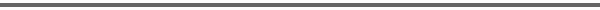Journal Home Page Complete Contentsof this Volume Previous Article Next Article Journal of Lie Theory 14 (2004), No. 2, 443--479 Copyright Heldermann Verlag 2004Hierarchy of Closures of Matrix Pencils Dmitri D. Pervouchine Dept. of Mathematics and Statistics, Boston University, 111 Cummington Street, Boston MA 02215, U.S.A., dp@bu.edu[Abstract-pdf] \def\GL{\mathop{\rm GL}} \def\SL{\mathop{\rm SL}} \def\C{\Bbb C}The focus of this paper is the standard linear representation of the group $\SL_n(\C)\times\SL_m(\C)\times\SL_2(\C)$, that is, the tensor product of the corresponding tautological representations. Classification of its orbits is a classic problem, which goes back to the works of Kronecker and Weierstrass. Here, we summarize some known results about standard linear representations of $\SL_n(\C)\times\SL_m(\C)\times\SL_2(\C)$, $\GL_n(\C)\times\GL_m(\C)$, $\SL_n(\C)\times\SL_m(\C)$, and $\GL_n(\C)\times\GL_m(\C)\times\GL_2(\C)$, classify the orbits and describe their degenerations. For the case $n\ne m$, we prove that the algebras of invariants of the actions of $\SL_n(\C)\times\SL_m(\C)\times\SL_2(\C)$ and $\SL_n(\C)\times\SL_m(\C)$ are generated by one polynomial of degree $nm/|n-m|$, if $d=|n-m|$ divides $n$ (or $m$), and are trivial otherwise. It is also shown that the null cone of $\SL_n(\C)\times\SL_m(\C)\times\SL_2(\C)$ is irreducible and contains an open orbit if $n\ne m$. We relate the degenerations of orbits of matrix pencils to the degenerations of matrix pencil bundles and prove that the closure of a matrix pencil bundle consists of closures of the corresponding orbits and closures of their amalgams. From this fact we derive the degenerations of orbits of the four groups listed above. All degenerations are cofined to the list of minimal degenerations, which are summarized as transformations of Ferrer diagrams. We tabulate the orbits of matrix pencils up to seventh order and portray the hierarchy of closures of $2\times2$, $3\times3$, $4\times4$, $5\times5$, $5\times6$ and $6\times6$ matrix pencil bundles. [FullText-pdf (325 KB)]] for subscribers only.